## LetsPlayMaths.Com

WELCOME TO THE WORLD OF MATHEMATICS

# Class 4 Fractions

Introduction to Fractions

Type of Fractions

Conversion of Fractions

Comparison of Fractions

Addition of Fractions

Subtraction of Fractions

Multiplication of Fractions

Reciprocal of Fractions

Division of Fractions

Fractions Test

Fractions Worksheet

Answer Sheet

## Introduction to Fractions

In previous class we learnt following things about fractions.

a) Meaning of Fractions

b) Meaning of Numerator and denominator

c) Equivalent Fractions

d) Comparison of fractions

e) Addition of fractions

f) Subtraction of fractions

A fraction represents a part of the whole. For example, 12 is a fraction, that means 1 part out of 2 equal parts.14 is a fraction, it denotes 1 part out of 4 equal parts.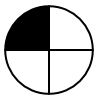38 is a fraction, it denotes 3 equal parts out of 8 equal parts. Here 3 is numerator and 8 is denominator.## Type of Fractions

There are 4 types of fractions and they are mentioned below.

a) Unit Fractions

b) Proper fractions

c) Improper fractions

d) Mixed fractions

Unit Fractions

Fractions which have 1 in their numerator are known as unit fractions. Few examples are given below.

12, 13, 14, 15, 19, 121, etc.

Proper Fractions

Fractions whose numerator is smaller than the denominator are known as proper fractions. Few examples are given below.

25, 57, 37, 712, etc.

Improper Fractions

Fractions whose numerator is greater than or equal to the denominator are known as improper fractions. Few examples are given below.

52, 74, 2527, 4321, etc.

Mixed Fractions

This type of fractions are combination of a whole number and a proper fraction. Few examples are given below.

112, 223, 357, 515, etc.

## Conversion of Fractions

We can convert improper fractions to mixed fractions and vice versa. Let's have a look at some examples provided below.

Conversion of Improper Fractions to Mixed Fractions

Improper fractions can be converted into mixed fractions by dividing the numerator by the denominator. The quotient gives the whole number part of the mixed fractions, and the remainder placed over the denominator gives the fractional part.

Example 1. Convert 103 into a mixed fraction.

Solution.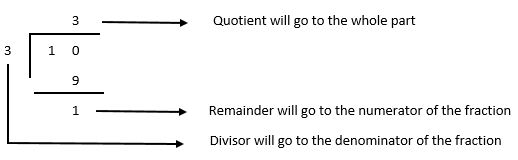So, the answer will be 313.

Example 2. Convert 11220 into a mixed fraction.

Solution.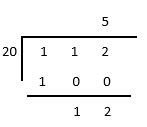Quotient 5 will be treated as whole number of mixed fraction.

Fraction part of the mixed fraction = 1220 = 35

So, the mixed fraction will be 535.

Conversion of Mixed Fractions into Improper Fractions

A mixed fraction can be changed into an improper fraction by multiplying the whole number by the denominator of the fraction and adding the product to numerator of the fraction.## Comparison of Fractions

Comparison of Like Fractions

If both the fractions denominators are same, then we compare the numerators of both the fractions. The fraction which has bigger numerator is the bigger fraction.

Example 1. Which of the fraction is smaller 35 or 25 ?

Solution. As both the fractions have same denominator, we must compare the numerators.

3 > 2. So, 35 > 25.

Example 2. Which of the fraction is smaller 10611 or 12311 ?

Solution. Both fractions numerator are same, that is 11. Now we need to compare the numerators.

123 > 106. So, 12311 > 10611.

Comparison of Unlike Fraction with Same Numerator

When the numerator of both the fractions are same, then the fraction which is having smaller denominator is the bigger fraction.

Example 1. Which of the following fraction is greater, 257 or 2517 ?

Solution. In this comparison both the fractions are having same numerator that is 25. Now, we need to compare the denominators of both the fractions.
Here 7 < 17, that means 257 > 2517.

Example 2. Which of the following fraction is greater, 105109 or 10589 ?

Solution. Both the fractions are having same numerator that is 105. Now, time to compare the denominators of both the fractions, 89 > 109.

So, 10589 > 105109.

Comparison of Unlike Fractions with Different Numerator and Different Denominator

When both the numerator and denominator of both fractions are different, then to find greater fraction we need to do following things. Let's consider 37 and 25 for comparison.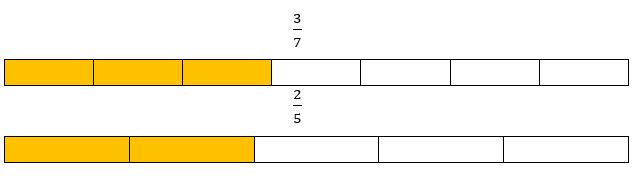Step-1
we multiply 1st fraction�s denominator with both the numerator and denominator of the 2nd fraction. 1st fraction denominator is 7, we have to multiply it to both numerator and denominator of 25.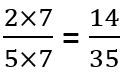Step-2
Similarly, we need to multiply 2nd fraction's denominator 5 with both numerator and denominator 37.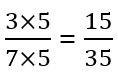Step-3
Now comparing 1435 and 1535 became easy as both fractions denominator became same. So, 1435 < 1535 or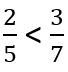Comparison of Mixed Fractions

For comparing mixed fractions, we have to check the whole numbers first, if the whole numbers are same then we have to compare the fractions.

Example 1. Compare 525 and 413.

Solution. Whole number of 525 is 5 and the whole number of 413 is 4.
5 > 4. So, 525 > 413.

Example 2. Compare 123 and 125.

Solution. Whole number of both the fractions are same here. We can not decide which fraction is bigger considering the whole number. Now, we need to check the fraction part, 23 and 25. Among these two fractions, 23 is bigger than 25.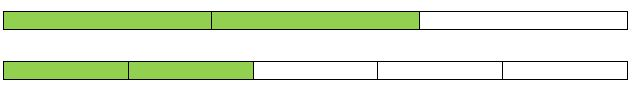So, 123 > 125.

## Addition of Fractions

In our previous class we learnt the basics of fraction addition. Here we will learn various types of fraction addition.

Addition of Fractions with Same Denominator

When both the fractions have same denominator, we first add the numerators and then place the sum over the common denominator. We simplify further if needed.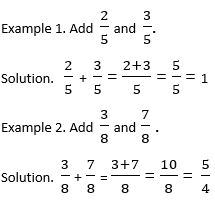Please have a look at the below given video for better understanding.

Addition of Fractions with different Denominators

In this kind of addition, we first find the LCM of all the denominators. Then convert all the fractions into equivalent fractions having common denominators as LCM. Then we add all the equivalent fractions. Let�s have a look at some examples.

Please have a look at the below given video for better understanding.

Example 1. Add 38 and 26.

Solution. LCM of 8 and 6 is 24.
To get the equivalent fraction of 38 whose denominator should be 24, we need to do the following.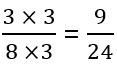Similarly, to get the equivalent fraction of 26 whose denominator should be 24, we need to do the following.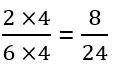Add both the equivalent fractions.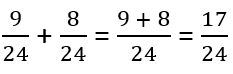So, the answer is 1724.

Addition of Mixed Fractions

First convert the mixed fractions into improper fractions and then add them as normal fractions rule. Let's have a look at some examples.

Example 1. Add 235 and 323.

Solution. 235 is equal to 135.
323 is equal to 113.Now, we have to follow the addition procedure by taking out the LCM of the denominators 5 and 3.
LCM of 5 and 3 = 15This can be done in another method.
First add the whole numbers, that is, 2 + 3 = 5 and keep it on the left side.
Now add the fractions 35 and 23 =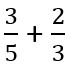Now, we have to follow the addition procedure by taking out the LCM of the denominators 5 and 3.
LCM of 5 and 3 = 15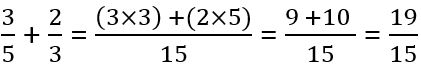We can further simplify 19/15 to mixed fraction = 1915 = 1415
We have whole numbers 5 which came from earlier whole numbers addition.
So, the total sum =Please visit below mentioned links for more fraction addition examples and worksheet.

Fraction Addition

## Subtraction of Fractions

In our previous class we learnt the basics of fraction subtraction. Here we will learn various types of fraction subtraction.

Subtraction of Fractions with Equal Denominator

To subtract fractions having equal denominator, we first subtract the numerators. Then we have to place the answer over the denominator. Let's have a look at some examples.

Please have a look at the below given video for better understanding.

Example 1. Subtract 25 from 45.

Solution.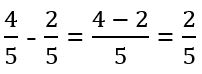Example 2. Subtract 257 from 357.

Solution.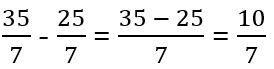Subtraction of Fractions with Different Denominators

To subtract fractions having different denominators, we first take out the LCM of the denominators. Then convert all the fractions into equivalent fractions having common denominators as LCM. Then we subtract equivalent fractions. Let's have a look at some examples.

Example 1. Subtract 34 from 78.

Solution. LCM of 4 and 8 is 8.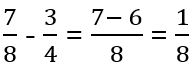Example 2. Subtract 525 from 35.

Solution. LCM of 5 and 25 is 25.1025 can be simplified further, that is, 25.
So, 25 is the answer.

Please have a look at the below given video for better understanding.

Subtraction of Mixed Fractions

In this kind of subtraction, first convert the mixed fractions into improper fractions and then subtract them as normal fractions rule. Let's have a look at some examples.

Example 1. Subtract 225 from 323.

Solution. Improper fraction of 225 =Improper fraction of 323 =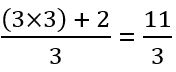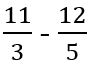LCM of 3 and 5 is 15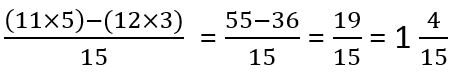So, the answer is 1415.

Subtraction from a Whole number

When we subtract a fraction from a whole number, we must convert the whole number to its fraction format. Then do the subtraction as per normal rules we learnt till now.

Example 1. Subtract 67 from 2.

Solution. Fraction format of 2 is 21.Now, subtract 67 from 21.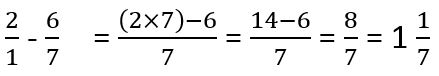So, the answer is 117.

## Multiplication of Fractions

Multiplication of Fraction by a Whole Number

To multiply a fraction by a whole number, we multiply the numerator of the fraction by the whole number and denominator of the fraction by 1. Let's have a look at some examples.

Example 1. Multiply 47 by 5.

Solution.So, the answer is 267.

Multiplication of Two Fractions

To multiply two fractions, we multiply the numerators of both the fractions and the product becomes numerator. Then, we multiply denominators of both the fractions and the product becomes denominator of the result. Let's have a look at some examples.

Example 1. Multiply 45 by 12.

Solution.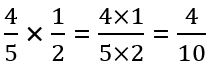If we simplify 410 further, then it will become 25.
So, the answer is 25.

Example 2. Multiply 225 by 323.

Solution. Here, we have two mixed fractions. First, we must convert them to improper fractions, then we do the multiplication.
Improper fraction of 225 = 125
Improper fraction of 323 = 113
Now multiply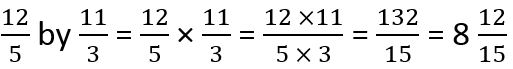So, the answer is 81215.

Please have a look at the below given video for better understanding.

## Reciprocal of Fractions

Two fractions whose product is 1 are called reciprocals. For example, 23 reciprocal is 32. If the product of two fractions is equal to 1 then we can say 23 and 32 are reciprocals.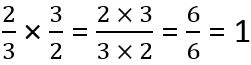In other words, if we want to find out the reciprocal of a fraction then interchange its numerator to denominator and
denominator to numerator.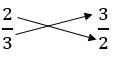Example 1. What is the reciprocal of 57?

Solution. Reciprocal of 57 is 75.

## Division of Fractions

In the division of fraction, we must find out the reciprocal of divisor and multiply it with the dividend. Let's have a look at some example.

Example 1. Divide 58 by 12.

Solution. 58 ÷ 12
Here, 58 is the dividend and 12 is divisor. Reciprocal of 12 is 21.So, the answer is 54.

Please have a look at the below given video for better understanding.

## Fractions Test

Fractions Test - 1

Fractions Test - 2

Fractions Test - 3

## Class-4 Fractions Worksheet

Fractions Worksheet - 1

Fractions Worksheet - 2

Fractions Worksheet - 3

Fractions Worksheet - 4

Fractions Worksheet - 5

Fractions Worksheet - 6

Fractions Worksheet - 7

Fractions Worksheet - 8

## Answer Sheet

Fractions-AnswerDownload the pdf

Copyright © 2021 LetsPlayMaths.com. All Rights Reserved.